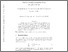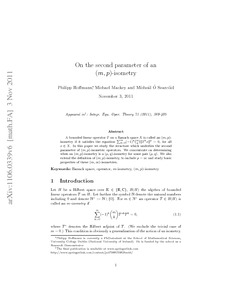# On the second parameter of an (m, p)-isometry

Hoffmann, Philipp and Mackey, Michael and Ó Searcóid, Mícheál (2011) On the second parameter of an (m, p)-isometry. Working Paper. arXiv.org.Previewmore...Add this article to your Mendeley library

## Abstract

A bounded linear operator T on a Banach space X is called an (m, p)-isometry if it satisfies the equation ∑mk=0(−1)k(mk)||p=0, for all x ∈ X. In this paper we study the structure which underlies the second parameter of (m, p)-isometric operators. We concentrate on determining when an (m, p)-isometry is a (μ, q)-isometry for some pair (μ, q). We also extend the definition of (m, p)-isometry, to include p = ∞ and study basic properties of these (m,∞)-isometries.

Item Type: Monograph (Working Paper) Preprint article available at DOI: 10.1007/s00020-011-1905-0 Cite as arXiv:1106.0339 [math.FA] Banach space; operator; m-isometry; (m, p)-isometry; Faculty of Science and Engineering > Mathematics and Statistics 5858 https://doi.org/10.1007/s00020-011-1905-0 Philipp Hoffmann 18 Feb 2015 15:34 arXiv.orgItem control page